NEET  >  32 Year NEET Previous Year Questions: Moving Charges & Magnetism - 1

# 32 Year NEET Previous Year Questions: Moving Charges & Magnetism - 1

Test Description

## 25 Questions MCQ Test Physics Class 12 | 32 Year NEET Previous Year Questions: Moving Charges & Magnetism - 1

32 Year NEET Previous Year Questions: Moving Charges & Magnetism - 1 for NEET 2023 is part of Physics Class 12 preparation. The 32 Year NEET Previous Year Questions: Moving Charges & Magnetism - 1 questions and answers have been prepared according to the NEET exam syllabus.The 32 Year NEET Previous Year Questions: Moving Charges & Magnetism - 1 MCQs are made for NEET 2023 Exam. Find important definitions, questions, notes, meanings, examples, exercises, MCQs and online tests for 32 Year NEET Previous Year Questions: Moving Charges & Magnetism - 1 below.
Solutions of 32 Year NEET Previous Year Questions: Moving Charges & Magnetism - 1 questions in English are available as part of our Physics Class 12 for NEET & 32 Year NEET Previous Year Questions: Moving Charges & Magnetism - 1 solutions in Hindi for Physics Class 12 course. Download more important topics, notes, lectures and mock test series for NEET Exam by signing up for free. Attempt 32 Year NEET Previous Year Questions: Moving Charges & Magnetism - 1 | 25 questions in 50 minutes | Mock test for NEET preparation | Free important questions MCQ to study Physics Class 12 for NEET Exam | Download free PDF with solutions
 1 Crore+ students have signed up on EduRev. Have you?
32 Year NEET Previous Year Questions: Moving Charges & Magnetism - 1 - Question 1

### When a proton is accelerated through 1 V, thenits kinetic energy will be 

Detailed Solution for 32 Year NEET Previous Year Questions: Moving Charges & Magnetism - 1 - Question 1

Potential difference (V) = 1V, K.E. acquired = qV = 1.6 × 10–19 × 1
= 1.6 × 10–19  joules = 1 eV

32 Year NEET Previous Year Questions: Moving Charges & Magnetism - 1 - Question 2

### If a long hollow copper pipe carries a current,then magnetic field is produced 

Detailed Solution for 32 Year NEET Previous Year Questions: Moving Charges & Magnetism - 1 - Question 2

Inside a hollow pipe carrying current, the magnetic field is zero, since according to Ampere's law, Bi. 2πr = μ0 × 0 ⇒ Bi = 0.
But for external points, the current behaves as if it was concentrated at the axis only; so, outside,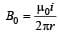Thus, the magnetic field is produced outside the pipe only.

32 Year NEET Previous Year Questions: Moving Charges & Magnetism - 1 - Question 3

### Two long parallel wires P and Q are both perpendicular to the plane of the paper with distance of 5 m between them. If P and Q carry currents of 2.5 amp and 5 amp respectively in the same direction, then the magnetic field at a point half-way between the wires is       

Detailed Solution for 32 Year NEET Previous Year Questions: Moving Charges & Magnetism - 1 - Question 3

When the current flows in both wires in the same direction then magnetic field at half way due to the wire P,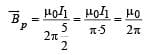(where I1= 2.5 amp) The direction of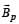is downward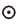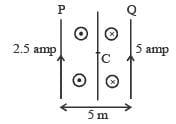Magnetic field at half way due to wire Q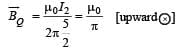[where I2 = 2.5amp.]

Net magnetic field at half way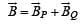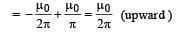Hence, net magnetic field at midpoint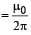32 Year NEET Previous Year Questions: Moving Charges & Magnetism - 1 - Question 4

A proton moving with a velocity 3 × 105 m/senters a magnetic field of 0.3 tesla at an angle of30º with the field. The radius of curvature of itspath will be (e/m for proton = 108 C/kg)         

Detailed Solution for 32 Year NEET Previous Year Questions: Moving Charges & Magnetism - 1 - Question 4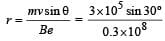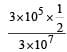= 0.5 x 10 -2m = 0.5cm

32 Year NEET Previous Year Questions: Moving Charges & Magnetism - 1 - Question 5

In a certain region of space electric field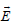and magnetic field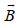are perpendicular to each other and an electron enters in region perpendicular to the direction ofandboth and moves undeflected, then velocity of electron is 

Detailed Solution for 32 Year NEET Previous Year Questions: Moving Charges & Magnetism - 1 - Question 5

Electron moves undeflected if force exerted due to electric field is equal to force due to magnetic field.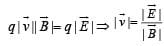32 Year NEET Previous Year Questions: Moving Charges & Magnetism - 1 - Question 6

A charged particle of charge q and mass m enters perpendicularly in a magnetic field. Kinetic energy of the particle is E; then frequency of rotation is 

Detailed Solution for 32 Year NEET Previous Year Questions: Moving Charges & Magnetism - 1 - Question 6

For circular path in magnetic field, mrω2 = qvB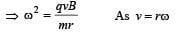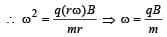∴  If v is frequency of roatation, then32 Year NEET Previous Year Questions: Moving Charges & Magnetism - 1 - Question 7

A galvanometer can be converted into avoltmeter by connecting           

Detailed Solution for 32 Year NEET Previous Year Questions: Moving Charges & Magnetism - 1 - Question 7

A galvanometer can be converted in to a voltmeter by connecting  the high ressistance in series with the galvanometer so that only a small amount of current passes through it.

32 Year NEET Previous Year Questions: Moving Charges & Magnetism - 1 - Question 8

A wire carries a current. Maintaining the samecurrent it is bent first to form a circular plane coil ofone turn which produces a magnetic field B at thecentre of the coil. The same length is now bentmore sharply to give a double loop of smaller radius.The magnetic field at the centre of the double loop,caused by the same current is          

Detailed Solution for 32 Year NEET Previous Year Questions: Moving Charges & Magnetism - 1 - Question 8

Let I be current and l be the length of the wire.
For Ist case :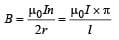where
2πr = l and n = 1

For IInd Case :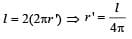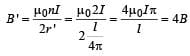32 Year NEET Previous Year Questions: Moving Charges & Magnetism - 1 - Question 9

A particle having charge q moves with a velocity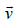through a region in which both an electric fieldand a magnetic fieldare present .The force on the particle is           

Detailed Solution for 32 Year NEET Previous Year Questions: Moving Charges & Magnetism - 1 - Question 9

Force due to electric field =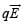Force due to magnetic field =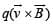Net force experienced =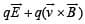32 Year NEET Previous Year Questions: Moving Charges & Magnetism - 1 - Question 10

A charged particle moves through a magneticfield in a direction perpendicular to it. Then the         

Detailed Solution for 32 Year NEET Previous Year Questions: Moving Charges & Magnetism - 1 - Question 10

Magnetic for ce acts perpendicular to the velocity. Hence speed remains constant.

32 Year NEET Previous Year Questions: Moving Charges & Magnetism - 1 - Question 11

A long solenoid carrying a current produces amagnetic field B along its axis. If the current isdouble and the number of turns per cm is halved,the new value of the magnetic field is     

Detailed Solution for 32 Year NEET Previous Year Questions: Moving Charges & Magnetism - 1 - Question 11

B = μ0ni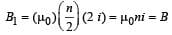⇒ B1 = B

32 Year NEET Previous Year Questions: Moving Charges & Magnetism - 1 - Question 12

A galvanometer acting as a voltmeter will have            

Detailed Solution for 32 Year NEET Previous Year Questions: Moving Charges & Magnetism - 1 - Question 12

A high resistance is connected in series so that less current passes through voltmeter.

32 Year NEET Previous Year Questions: Moving Charges & Magnetism - 1 - Question 13

A galvanometer of 50 ohm resistance has 25 divisions. A current of 4 × 10–4 ampere gives adeflection of one per division. To convert thisgalvanometer into a voltmeter having a rangeof 25 volts, it should be connected with aresistance of 

Detailed Solution for 32 Year NEET Previous Year Questions: Moving Charges & Magnetism - 1 - Question 13

Rg = 50Ω, Ig = 25 × 4 × 10–AΩ = 10–2 A
Range of V = 25 volts V = Ig(Re + Rg)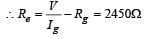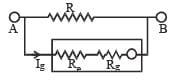32 Year NEET Previous Year Questions: Moving Charges & Magnetism - 1 - Question 14

An electron moves in a circular orbit with a uniform speed v. It produces a magnetic field B at the centre of the circle. The radius of the circle is proportional to      

Detailed Solution for 32 Year NEET Previous Year Questions: Moving Charges & Magnetism - 1 - Question 14

The magnetic field produce by moving electron in circular path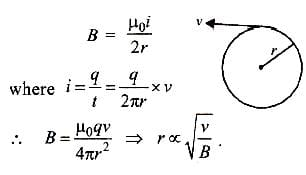32 Year NEET Previous Year Questions: Moving Charges & Magnetism - 1 - Question 15

A very long straight wire carries a current I. At the instant when a charge + Q at point P has velocity, as shown, the force on the charge is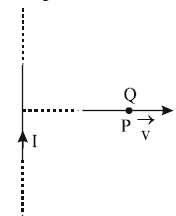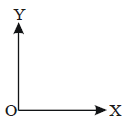Detailed Solution for 32 Year NEET Previous Year Questions: Moving Charges & Magnetism - 1 - Question 15

The direction of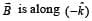∴  The magnetic  force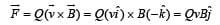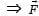is along OY.

32 Year NEET Previous Year Questions: Moving Charges & Magnetism - 1 - Question 16

When a charged particle moving with velocityis subjected to a magnetic field of inductionthe force on it is non-zero. This implies that

Detailed Solution for 32 Year NEET Previous Year Questions: Moving Charges & Magnetism - 1 - Question 16

Force on a particle moving with velocity v in a magnetic field  B is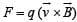If angle between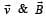is either zero or 180º, then value of F will be zero as cross product of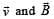will be zero.
So option (b) is correct.

32 Year NEET Previous Year Questions: Moving Charges & Magnetism - 1 - Question 17

Two circular coils 1 and 2 are made from the samewire but the radius of the 1st coil is twice that ofthe 2nd coil. What potential difference in voltsshould be applied across them so that themagnetic field at their centres is the same 

Detailed Solution for 32 Year NEET Previous Year Questions: Moving Charges & Magnetism - 1 - Question 17

If R1 & R2 be the radius of the circular wires,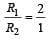If same potential is applied on them, current in Ist will be half that in the later. If V potential is applied on them, current in them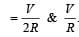Now magnetic field at the centre of circular

coil,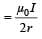For first wire, field B1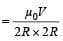For second wire, field B2  =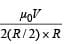Given B1 = B2 The given data do not provide any required result. There is a mistake in the framing of the question.

32 Year NEET Previous Year Questions: Moving Charges & Magnetism - 1 - Question 18

A beam of electron passes undeflected throughmutually perpendicular electric and magnetic fields.If the electric field is switched off, and the samemagnetic field is maintained, the electrons move  

Detailed Solution for 32 Year NEET Previous Year Questions: Moving Charges & Magnetism - 1 - Question 18

If the electric field is switched off, and the same magnetic field is maintained, the electrons move in a circular orbit and electron will travel a magnetic field ⊥ to its velocity..

32 Year NEET Previous Year Questions: Moving Charges & Magnetism - 1 - Question 19

In a mass spectrometer used for measuring the masses of ions, the ions are initially accelerated by an electric potential V and then made to describe semicircular path of radius R using a magnetic field B. If V and B are kept constant, the ratio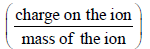will be proportional to

Detailed Solution for 32 Year NEET Previous Year Questions: Moving Charges & Magnetism - 1 - Question 19

In mass spectro meter, when ions are accelerated through potential V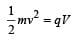..........(i)

As the magnetic field curves the path of the ions in a semicircular orbit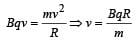.......... (ii)

Substituting (ii) in (i)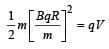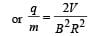Since V and B are constants,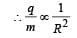32 Year NEET Previous Year Questions: Moving Charges & Magnetism - 1 - Question 20

Under the influence of a uniform magnetic fielda charged particle is moving in a circle of radiusR with constant speed v. The time period of themotion        

Detailed Solution for 32 Year NEET Previous Year Questions: Moving Charges & Magnetism - 1 - Question 20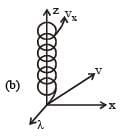When a test charge q0 enters a magnetic field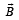directed along z-axis, with a velocity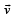making angles d with the z-axis. The time period of the motion is independent of R and v.

32 Year NEET Previous Year Questions: Moving Charges & Magnetism - 1 - Question 21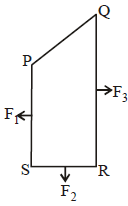A closed loop PQRS carrying a current is placed in a uniform magnetic field. If the magnetic forces on segments PS, SR, and RQ are F1 , F2 and F3 respectively and are in the plane of the paper and along the directions shown, the force on the segment QP is       

Detailed Solution for 32 Year NEET Previous Year Questions: Moving Charges & Magnetism - 1 - Question 21

According to the figure the magnitude of force on the segment QM is F3 –F1 and PM is F2.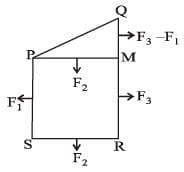Therefore, the magnitude of the force on segment PQ is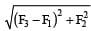32 Year NEET Previous Year Questions: Moving Charges & Magnetism - 1 - Question 22

A particle of mass m, charge Q and kinetic energyT enters a transverse uniform magnetic field ofinduction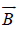. After 3 seconds, the kinetic energyof the particle will be: 

Detailed Solution for 32 Year NEET Previous Year Questions: Moving Charges & Magnetism - 1 - Question 22

When a charged particle enters a transverse magnetic field it traverse a circular path. Its kinetic energy remains constant.

32 Year NEET Previous Year Questions: Moving Charges & Magnetism - 1 - Question 23

A circular disc of radius 0.2 meter is placed in a uniform magnetic field of induction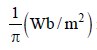in such a way that its axis makes an angle of 60° with. The magnetic flux linked with the disc is:        

Detailed Solution for 32 Year NEET Previous Year Questions: Moving Charges & Magnetism - 1 - Question 23

Here,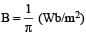θ = 60°
Area normal to the plane of the disc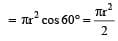Flux = B × normal area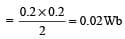32 Year NEET Previous Year Questions: Moving Charges & Magnetism - 1 - Question 24

A galvanometer having a coil resistance of 60 Ωshows full scale deflection when a current of 1.0amp passes through it. It can be convertedinto an ammeter to read currents upto 5.0 amp by         

Detailed Solution for 32 Year NEET Previous Year Questions: Moving Charges & Magnetism - 1 - Question 24

G = 60Ω, Ig=1.0A, I=5A.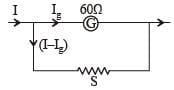Let S be the shunt resistance connected in parallel to galvanometer IgG = (I – Ig) S,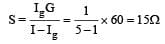Thus by putting 15Ω in parallel, the galvanometer can be converted into an ammeter.

32 Year NEET Previous Year Questions: Moving Charges & Magnetism - 1 - Question 25

A charged paritcle (charge q) is moving in a circleof radius R with uniform speed v. The associatedmagnetic moment μ is given by 

Detailed Solution for 32 Year NEET Previous Year Questions: Moving Charges & Magnetism - 1 - Question 25

Magnetic moment, m = IA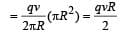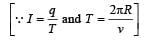## Physics Class 12

157 videos|452 docs|213 tests
 Use Code STAYHOME200 and get INR 200 additional OFF Use Coupon Code
Information about 32 Year NEET Previous Year Questions: Moving Charges & Magnetism - 1 Page
In this test you can find the Exam questions for 32 Year NEET Previous Year Questions: Moving Charges & Magnetism - 1 solved & explained in the simplest way possible. Besides giving Questions and answers for 32 Year NEET Previous Year Questions: Moving Charges & Magnetism - 1, EduRev gives you an ample number of Online tests for practice

## Physics Class 12

157 videos|452 docs|213 tests

### How to Prepare for NEET

Read our guide to prepare for NEET which is created by Toppers & the best Teachers